|

# 暗卫通风,你以为选个大功率的排风扇就行了

有人说，卫生间通风有什么好详解的，买个大功率的排气扇就行了，又想骗流量是吧。但凡是这么说的，都是还在租房的，因为没装过修，他们忽略了一个前提。

我们想一下，暗卫通风的目的是什么——除湿去味；那再往下想一步，为什么会有湿气和味道，因为没有窗……这是一个客观原因，还有主观原因——防水与漏水没做好！

这也是为什么说暗卫通风要从防水开始；暗卫通风实际上是要解决卫生间内的潮湿与有异味的问题，在观念上大家要扭过来，不要以为只装个排气扇就万事大吉了。因而，文章我们用两部分来讲：1、怎么解决卫生间的潮湿问题；2、怎么有效的预防与去除暗卫的异味。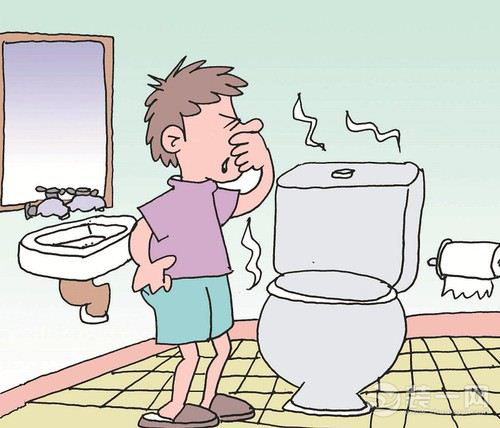一、怎么解决卫生间的潮湿问题

卫生间潮湿是一定的，特别是暗卫，早晨洗脸要用水，晚上洗澡要用水，不通风的话，肯定是潮潮的，时间久了容易滋生细菌，产生异味。

因而，解决卫生间潮湿问题，首先要做好防水，这是一个极其重要的基础工程，要注意的是淋浴喷头两侧墙面要做到1.8米高的防水，面池区1.2米，其余上反0.3米，以防止水浸透墙面，造成墙面发霉，地面则要全面做防水。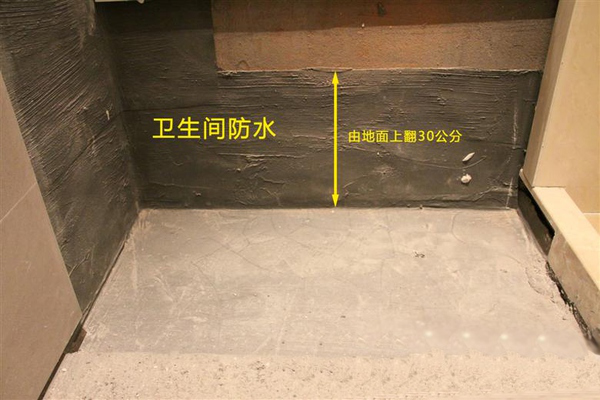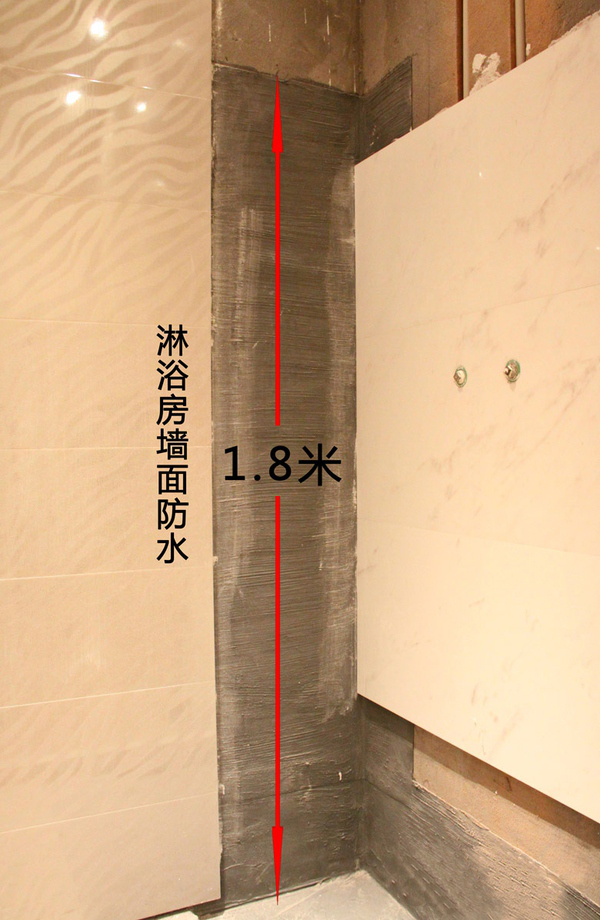其次，做好干湿分离，你可以使用便宜又好装的浴帘；在淋浴区设置坡度，使水流向地漏；安一个小门槛，专业说法是防水条，阻挡水的流出。不影响光线的玻璃隔断、半隔断/全隔断；和浴帘一样，做地面坡度、水流向地漏，或者干脆安装下水更快的长条地漏；安防水条，把水暂时困在里面。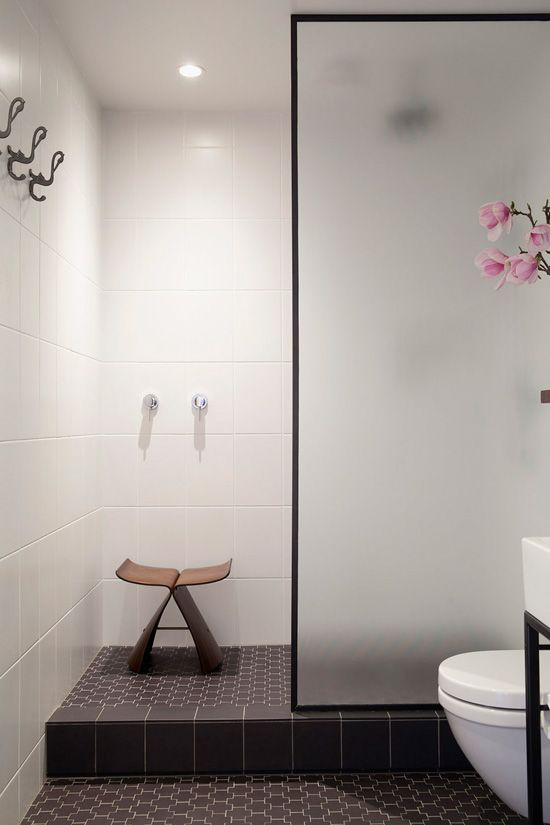解决了隔水的问题，半隔断玻璃墙的优势就更明显了：相比于其他隔断，它让你的视野更加通透、而且洗澡不闷，尤其适合小卫生间。

更具空间感的隔断墙：如果你的卫生间足够大，也可以考虑使用隔断墙。墙会使浴室更有空间感，更能保护隐私，还可以在墙上做收纳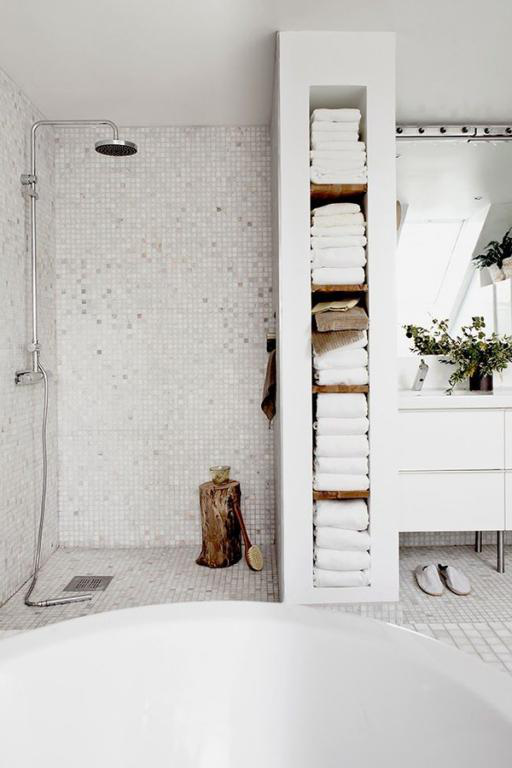再次，也是绕不开的，你需要一个换气量大的排风扇。

为什么不是功率大，而是换气量大，因为在功率相同的情况下，噪音是相同的，而换气量更大的排风扇能更快的把卫生间的污浊空气排出。

那怎么选排风扇的换气量：换气量的国家标准是卫生间是每小时15次，也就是说你家卫生间长3m*宽3m*高2.4m*15，需要的是风量为324立方米/时的排风扇，实际上需要更大一些的风量，因为管道回风会损失一些。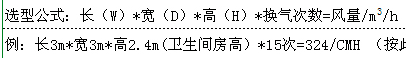除此之外，排风扇的选购尽量选择顶吸式的，而不要选悬挂式，窗口式的就不提了（连窗都没有），原因在于悬挂式排风效果较差，对暗卫来说，需要的是大风量的排气扇。可选择的品牌很多，奥普、松下、正野、艾美特、雷士等，其中松下和奥普是比较好的选择，松下排风扇的优点是：与其他品牌的排风扇相比，在相同功率下，风量更大，噪音较小；但价格相对较高。奥普则是国产品牌里做的相对较好的。

需要注意的是，如果排风扇没有止逆阀，则要在通风管道里加上止逆阀，防止通风管道产生异味。

二、如何有效的预防与去除暗卫的异味

我们首先要弄清楚的是卫生间产生异味的源头在那里，在我看来源头有五个：地漏产生异味、洗面盆下水管产生异味、坐便器产生异味、通风道排风扇产生异味。

我们分别来说解决办法：

1、地漏产生异味，很大程度上是地漏本身造成的。其储水弯深度不够，气味往上冒，是其中最大的原因；此外，毛发堵塞变质有味也是常见的因素。

也就是说，买好了地漏就解决了这个问题。现在市场上所说的防臭地漏，宣传的漏芯都是硅胶什么的材料，其实真的有用么，不见得。地漏的防臭原理并不在于材料，而在于水深（至少50mm），面板大小有长*宽100mm*100mm和1200m*120mm的，可根据需求选购。

（以水封地漏的原理为例）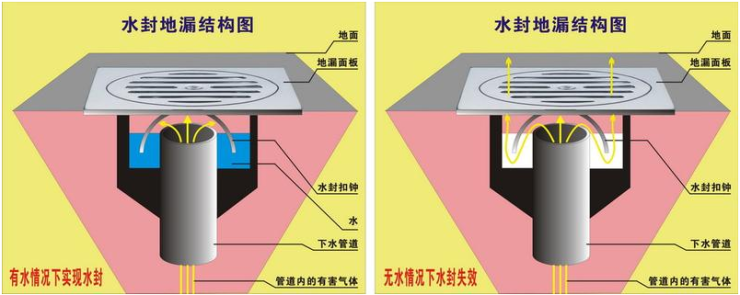储水弯的水才能防止下水管道反味，也就是说储水弯的深度是最关键的。现在市面上麦的地漏类型常见的有以几种，三防地漏是较好的；至于宣传的四防、六防、九防地漏，更多的是在一些功能上的健全，但远没有那么邪乎，且防的越多下水可能越不畅。选购地漏时，需要注意的是储水弯深度（至少50mm）；面板材质（铜镀铬好，铜制较好，不锈钢次之，合金较差，易生锈）；下水快（不要细小圆孔的，毛发容易堵塞）；不返水（主要看储水弯的设计，U型和V型地漏有较好的防反水功能）。

如潜水艇的U型地漏：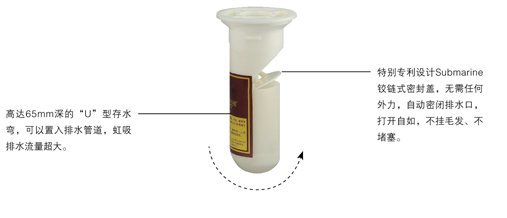说再多也不如实实在在的推荐几个好的地漏产品：

现在市场上的地漏产品很多，鱼龙混杂，但还是有一些品牌值得信赖，如潜水艇、邦哥、坦克防臭地漏、新润爽、九牧等；其中潜水艇是特别推荐的，虽然价格稍贵，但东西确实好，马云家和奶茶家都有卖，大家可以根据规格和价格及评价去选。

图为京东卖的较好的四款潜水艇的产品。2、马桶产生异味，这个问题现在已经很少了，只需要选择一款好点的马桶，然后正确的安装就行了。

若选卫洗丽的话请点：吃土有保障，剁手有指南之卫洗丽

若选智能马桶的话点：选购智能马桶 重点关注哪些功能？

若选一般马桶的话，我们来说下该注意哪些点，以及有哪些品牌是可以选的。

注意的点：坑距，也就是马桶下水到墙面的距离，准确说是地面下水孔中心点距未装修墙面的距离，这关系到选购马桶的大小，太大的话需要改动下水管，费事费钱。

选购之前，只需要注意这一点就行了。可选的品牌：

我说再多关于马桶釉面、冲水方式、水箱容量、水件好坏的数据，你也不会留意太多，还不如直接说那些可以选的品牌的产品。

若选陶瓷马桶，就是杜拉维特的最好，其次TOTO、科勒，然后是中宇、箭牌、法恩莎，若是排水和隐蔽水箱的话，吉博力的好。

3、排风管道的异味可以用止逆阀来解决，现在市面上很多排风扇本身就带有止逆阀，若没有的话，就装一个。有时开发商会在毛坯房上装一个铁质的止逆阀，但大多会换成塑料的，铁质的会生锈。至于，洗脸盆下的水管反味问题，你需要一个防臭的软管，如下图：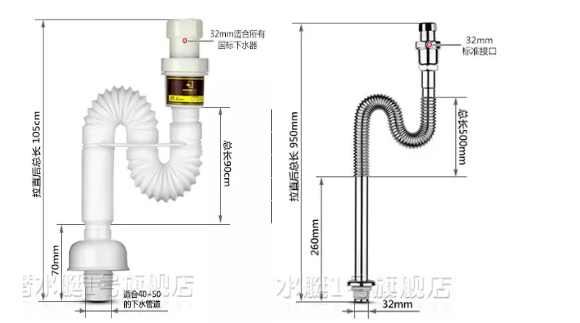S型的构造，可以有效的储水，防止脏水味道上翻；内置的防臭阀门，在有水下流的时候，才会打开，其余时间是闭合的。材质铜制的较好，塑料的次之。

品牌：潜水艇最好、卡贝次之、再次之是九牧。

还有C型的，九牧有，但防反味效果并不理想。总之，暗卫通风是一个系统，并非是一个排风扇就能解决的。从装修伊始，就要考虑这个问题，从防水到干湿区分割，再到除湿，都是要做好的。如果，你特别不喜欢潮湿，你可能还需要增加一个抽湿机。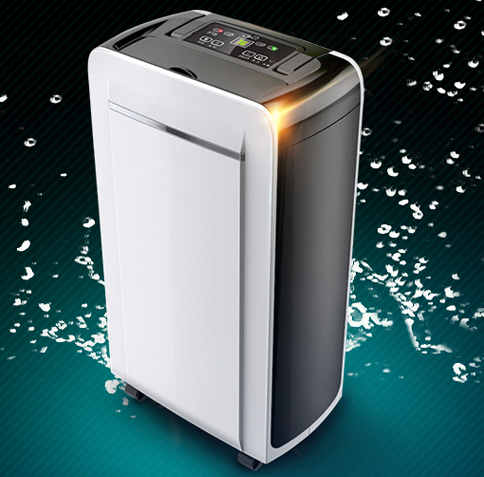最后，在这里手动打个广告，住好家开通了微信订阅号，大家可以搜索【住好家指南】，我们有专业的设计师可以给大家提供装修建议，只要你提供需求，我们可以给您家免费进行装修方案评估和主材预算评估，并且承诺在24小时完成评估报告，有兴趣的可以添加我们的公众号哦~~

声明：近期文章推荐主材选择，涉及到一些品牌，只是根据网友真实反馈进行说明，所述仅代表【住好家】品牌设计师和家居编辑个人观点，绝对不存在给任何品牌做广告的嫌疑！

（本文为住好家原创，欢迎转发分享）

`声明：本文由入驻焦点开放平台的作者撰写，除焦点官方账号外，观点仅代表作者本人，不代表焦点立场错误信息举报电话： 400-099-0099，邮箱：jubao@vip.sohu.com，或点此进行意见反馈，或点此进行举报投诉。`A B C D E F G H J K L M N P Q R S T W X Y Z
A - B - C - D - E
• A
• 鞍山
• 安庆
• 安阳
• 安顺
• 安康
• 澳门
• B
• 北京
• 保定
• 包头
• 巴彦淖尔
• 本溪
• 蚌埠
• 亳州
• 滨州
• 北海
• 百色
• 巴中
• 毕节
• 保山
• 宝鸡
• 白银
• 巴州
• C
• 承德
• 沧州
• 长治
• 赤峰
• 朝阳
• 长春
• 常州
• 滁州
• 池州
• 长沙
• 常德
• 郴州
• 潮州
• 崇左
• 重庆
• 成都
• 楚雄
• 昌都
• 慈溪
• 常熟
• D
• 大同
• 大连
• 丹东
• 大庆
• 东营
• 德州
• 东莞
• 德阳
• 达州
• 大理
• 德宏
• 定西
• 儋州
• 东平
• E
• 鄂尔多斯
• 鄂州
• 恩施
F - G - H - I - J
• F
• 抚顺
• 阜新
• 阜阳
• 福州
• 抚州
• 佛山
• 防城港
• G
• 赣州
• 广州
• 桂林
• 贵港
• 广元
• 广安
• 贵阳
• 固原
• H
• 邯郸
• 衡水
• 呼和浩特
• 呼伦贝尔
• 葫芦岛
• 哈尔滨
• 黑河
• 淮安
• 杭州
• 湖州
• 合肥
• 淮南
• 淮北
• 黄山
• 菏泽
• 鹤壁
• 黄石
• 黄冈
• 衡阳
• 怀化
• 惠州
• 河源
• 贺州
• 河池
• 海口
• 红河
• 汉中
• 海东
• 怀来
• I
• J
• 晋中
• 锦州
• 吉林
• 鸡西
• 佳木斯
• 嘉兴
• 金华
• 景德镇
• 九江
• 吉安
• 济南
• 济宁
• 焦作
• 荆门
• 荆州
• 江门
• 揭阳
• 金昌
• 酒泉
• 嘉峪关
K - L - M - N - P
• K
• 开封
• 昆明
• 昆山
• L
• 廊坊
• 临汾
• 辽阳
• 连云港
• 丽水
• 六安
• 龙岩
• 莱芜
• 临沂
• 聊城
• 洛阳
• 漯河
• 娄底
• 柳州
• 来宾
• 泸州
• 乐山
• 六盘水
• 丽江
• 临沧
• 拉萨
• 林芝
• 兰州
• 陇南
• M
• 牡丹江
• 马鞍山
• 茂名
• 梅州
• 绵阳
• 眉山
• N
• 南京
• 南通
• 宁波
• 南平
• 宁德
• 南昌
• 南阳
• 南宁
• 内江
• 南充
• P
• 盘锦
• 莆田
• 平顶山
• 濮阳
• 攀枝花
• 普洱
• 平凉
Q - R - S - T - W
• Q
• 秦皇岛
• 齐齐哈尔
• 衢州
• 泉州
• 青岛
• 清远
• 钦州
• 黔南
• 曲靖
• 庆阳
• R
• 日照
• 日喀则
• S
• 石家庄
• 沈阳
• 双鸭山
• 绥化
• 上海
• 苏州
• 宿迁
• 绍兴
• 宿州
• 三明
• 上饶
• 三门峡
• 商丘
• 十堰
• 随州
• 邵阳
• 韶关
• 深圳
• 汕头
• 汕尾
• 三亚
• 三沙
• 遂宁
• 山南
• 商洛
• 石嘴山
• T
• 天津
• 唐山
• 太原
• 通辽
• 铁岭
• 泰州
• 台州
• 铜陵
• 泰安
• 铜仁
• 铜川
• 天水
• 天门
• W
• 乌海
• 乌兰察布
• 无锡
• 温州
• 芜湖
• 潍坊
• 威海
• 武汉
• 梧州
• 渭南
• 武威
• 吴忠
• 乌鲁木齐
X - Y - Z
• X
• 邢台
• 徐州
• 宣城
• 厦门
• 新乡
• 许昌
• 信阳
• 襄阳
• 孝感
• 咸宁
• 湘潭
• 湘西
• 西双版纳
• 西安
• 咸阳
• 西宁
• 仙桃
• 西昌
• Y
• 运城
• 营口
• 盐城
• 扬州
• 鹰潭
• 宜春
• 烟台
• 宜昌
• 岳阳
• 益阳
• 永州
• 阳江
• 云浮
• 玉林
• 宜宾
• 雅安
• 玉溪
• 延安
• 榆林
• 银川
• Z
• 张家口
• 镇江
• 舟山
• 漳州
• 淄博
• 枣庄
• 郑州
• 周口
• 驻马店
• 株洲
• 张家界
• 珠海
• 湛江
• 肇庆
• 中山
• 自贡
• 资阳
• 遵义
• 昭通
• 张掖
• 中卫

1室1厅1厨1卫1阳台

1
2
3
4
5

0
1
2

1

1

0
1
2
3报名成功，资料已提交审核A B C D E F G H J K L M N P Q R S T W X Y Z
A - B - C - D - E
• A
• 鞍山
• 安庆
• 安阳
• 安顺
• 安康
• 澳门
• B
• 北京
• 保定
• 包头
• 巴彦淖尔
• 本溪
• 蚌埠
• 亳州
• 滨州
• 北海
• 百色
• 巴中
• 毕节
• 保山
• 宝鸡
• 白银
• 巴州
• C
• 承德
• 沧州
• 长治
• 赤峰
• 朝阳
• 长春
• 常州
• 滁州
• 池州
• 长沙
• 常德
• 郴州
• 潮州
• 崇左
• 重庆
• 成都
• 楚雄
• 昌都
• 慈溪
• 常熟
• D
• 大同
• 大连
• 丹东
• 大庆
• 东营
• 德州
• 东莞
• 德阳
• 达州
• 大理
• 德宏
• 定西
• 儋州
• 东平
• E
• 鄂尔多斯
• 鄂州
• 恩施
F - G - H - I - J
• F
• 抚顺
• 阜新
• 阜阳
• 福州
• 抚州
• 佛山
• 防城港
• G
• 赣州
• 广州
• 桂林
• 贵港
• 广元
• 广安
• 贵阳
• 固原
• H
• 邯郸
• 衡水
• 呼和浩特
• 呼伦贝尔
• 葫芦岛
• 哈尔滨
• 黑河
• 淮安
• 杭州
• 湖州
• 合肥
• 淮南
• 淮北
• 黄山
• 菏泽
• 鹤壁
• 黄石
• 黄冈
• 衡阳
• 怀化
• 惠州
• 河源
• 贺州
• 河池
• 海口
• 红河
• 汉中
• 海东
• 怀来
• I
• J
• 晋中
• 锦州
• 吉林
• 鸡西
• 佳木斯
• 嘉兴
• 金华
• 景德镇
• 九江
• 吉安
• 济南
• 济宁
• 焦作
• 荆门
• 荆州
• 江门
• 揭阳
• 金昌
• 酒泉
• 嘉峪关
K - L - M - N - P
• K
• 开封
• 昆明
• 昆山
• L
• 廊坊
• 临汾
• 辽阳
• 连云港
• 丽水
• 六安
• 龙岩
• 莱芜
• 临沂
• 聊城
• 洛阳
• 漯河
• 娄底
• 柳州
• 来宾
• 泸州
• 乐山
• 六盘水
• 丽江
• 临沧
• 拉萨
• 林芝
• 兰州
• 陇南
• M
• 牡丹江
• 马鞍山
• 茂名
• 梅州
• 绵阳
• 眉山
• N
• 南京
• 南通
• 宁波
• 南平
• 宁德
• 南昌
• 南阳
• 南宁
• 内江
• 南充
• P
• 盘锦
• 莆田
• 平顶山
• 濮阳
• 攀枝花
• 普洱
• 平凉
Q - R - S - T - W
• Q
• 秦皇岛
• 齐齐哈尔
• 衢州
• 泉州
• 青岛
• 清远
• 钦州
• 黔南
• 曲靖
• 庆阳
• R
• 日照
• 日喀则
• S
• 石家庄
• 沈阳
• 双鸭山
• 绥化
• 上海
• 苏州
• 宿迁
• 绍兴
• 宿州
• 三明
• 上饶
• 三门峡
• 商丘
• 十堰
• 随州
• 邵阳
• 韶关
• 深圳
• 汕头
• 汕尾
• 三亚
• 三沙
• 遂宁
• 山南
• 商洛
• 石嘴山
• T
• 天津
• 唐山
• 太原
• 通辽
• 铁岭
• 泰州
• 台州
• 铜陵
• 泰安
• 铜仁
• 铜川
• 天水
• 天门
• W
• 乌海
• 乌兰察布
• 无锡
• 温州
• 芜湖
• 潍坊
• 威海
• 武汉
• 梧州
• 渭南
• 武威
• 吴忠
• 乌鲁木齐
X - Y - Z
• X
• 邢台
• 徐州
• 宣城
• 厦门
• 新乡
• 许昌
• 信阳
• 襄阳
• 孝感
• 咸宁
• 湘潭
• 湘西
• 西双版纳
• 西安
• 咸阳
• 西宁
• 仙桃
• 西昌
• Y
• 运城
• 营口
• 盐城
• 扬州
• 鹰潭
• 宜春
• 烟台
• 宜昌
• 岳阳
• 益阳
• 永州
• 阳江
• 云浮
• 玉林
• 宜宾
• 雅安
• 玉溪
• 延安
• 榆林
• 银川
• Z
• 张家口
• 镇江
• 舟山
• 漳州
• 淄博
• 枣庄
• 郑州
• 周口
• 驻马店
• 株洲
• 张家界
• 珠海
• 湛江
• 肇庆
• 中山
• 自贡
• 资阳
• 遵义
• 昭通
• 张掖
• 中卫• 手机• 分享
• 设计
免费设计
• 计算器
装修计算器
• 入驻
合作入驻
• 联系
联系我们
• 置顶
返回顶部